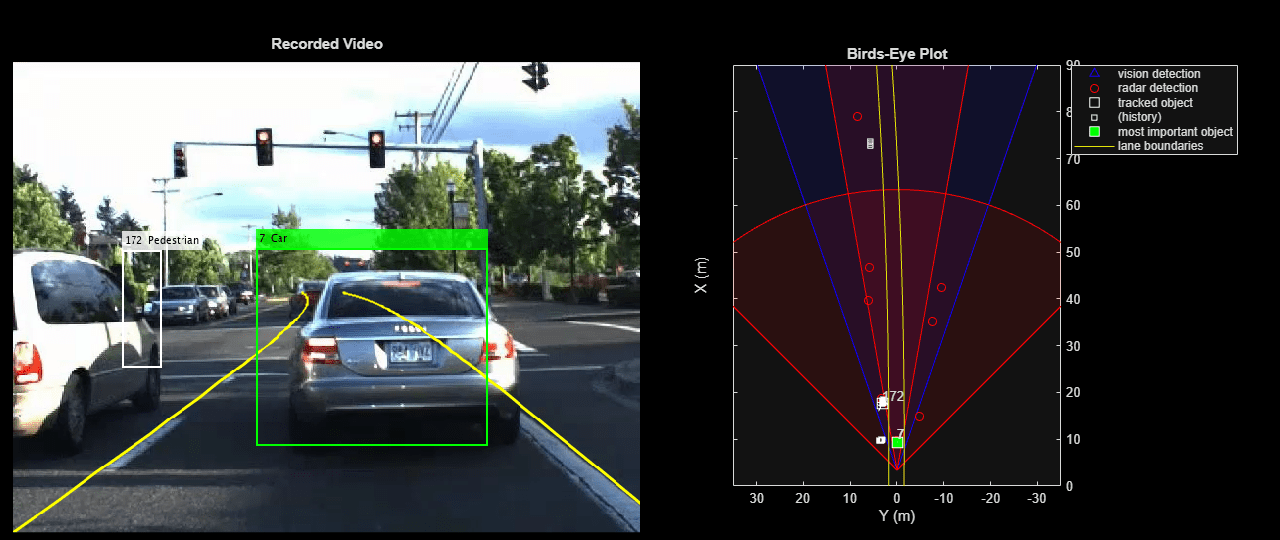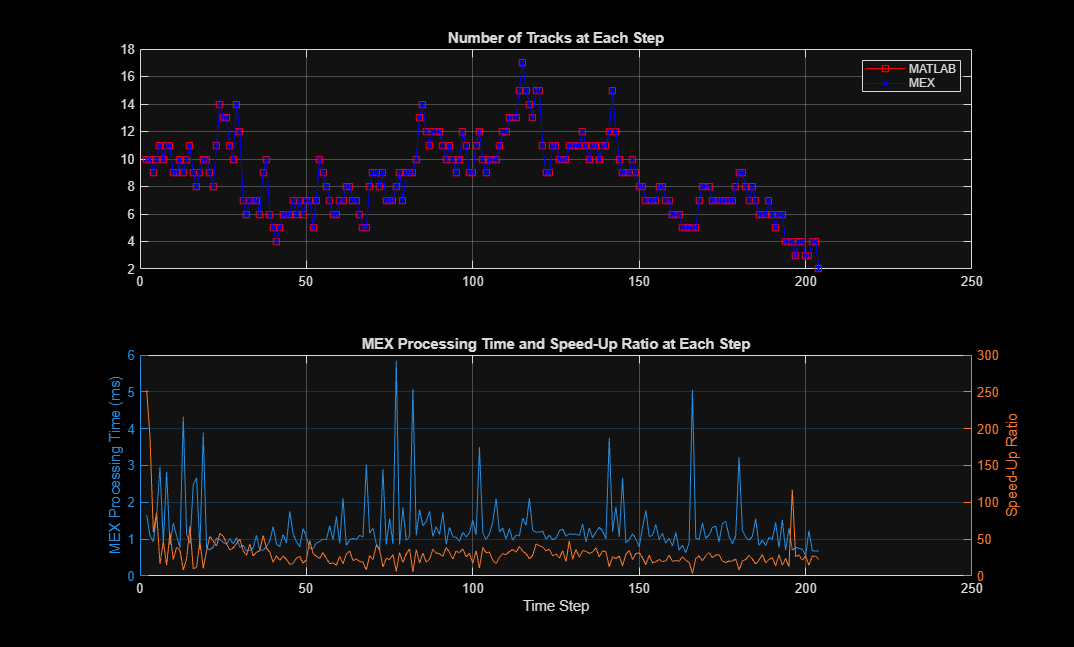# Code Generation for Tracking and Sensor Fusion

This example shows how to generate C code for a MATLAB function that processes data recorded from a test vehicle and tracks the objects around it.

Automatic generation of code from MATLAB code has two key benefits:

1. Prototypes can be developed and debugged in the MATLAB environment. Once the MATLAB work is done, automatic C code generation makes the algorithms deployable to various targets. Additionally, the C code can be further tested by running the compiled MEX file in a MATLAB environment using the same visualization and analysis tools that were available during the prototyping phase.

2. After generating C code, you can generate executable code, which in many cases runs faster than the MATLAB code. The improved run time can be used to develop and deploy real-time sensor fusion and tracking systems. It also provides a better way to batch test the tracking systems on a large number of data sets.

The example explains how to modify the MATLAB code in the Forward Collision Warning Using Sensor Fusion example to support code generation.

This example requires a MATLAB® Coder™ license for generating C code.

### Modify and Run MATLAB Code

You can learn about the basics of code generation using MATLAB Coder from the Introduction to Code Generation with Feature Matching and Registration example.

To generate C code, MATLAB Coder requires MATLAB code to be in the form of a function. Furthermore, the arguments of the function cannot be MATLAB classes.

In this example, the code for the forward collision warning (FCW) example has been restructured such that the functions that perform sensor fusion and tracking reside in a separate file, called `trackingForFCW_kernel.m`. Review this file for important information about memory allocation for code generation.

To preserve the state of the `multiObjectTracker` between calls to `trackingForFCW_kernel.m`, the tracker is defined as a `persistent` variable.

This function takes as an input a frame of the recorded data that includes:

1. Vision objects - A `struct` that contains 10 vision objects.

2. Radar objects - A `struct` that contains 36 radar objects.

3. Inertial measurement - A `struct` containing speed and yaw rate.

4. Lane reports - A `struct` array with parameters for the left and right lane boundaries.

Similarly, the outputs from a function that supports code generation cannot be objects. The outputs from `trackingForFCW_kernel.m` are:

1. Confirmed tracks - A `struct` array that contains a variable number of tracks.

2. Ego lane - A `struct` array with the parameters of left and right lane boundaries.

3. Number of tracks - An integer scalar.

4. Information about the most important object (MIO) and warning level from the FCW logic.

By restructuring the code this way, you can reuse the same display tools used in the FCW example. These tools still run in MATLAB and do not require code generation.

Run the following lines of code to load the recorded data and prepare the display in a similar way to the FCW example.

```% If a previous tracker is defined, clear it clear trackingForFCW_kernel % Set up the display videoFile = '01_city_c2s_fcw_10s.mp4'; sensorConfigFile = 'SensorConfigurationData.mat'; [videoReader, videoDisplayHandle, bepPlotters, sensor] = helperCreateFCWDemoDisplay(videoFile,sensorConfigFile); % Read the recorded detections file detfile = '01_city_c2s_fcw_10s_sensor.mat'; [visionObjects, radarObjects, imu, lanes, timeStep, numSteps] = helperReadSensorRecordingsFile(detfile); % An initial ego lane is calculated. If the recorded lane information is % invalid, define the lane boundaries as straight lines half a lane % distance on each side of the car. laneWidth = 3.6; % meters egoLane = struct('left', [0 0 laneWidth/2], 'right', [0 0 -laneWidth/2]); % Prepare some time variables timeStamp = 0; % Time since the beginning of the recording index = 0; % Index into the recorded sensor data % Define the position and velocity selectors: % The state vector is in constant acceleration: [x;vx;ax;y;vy;ay] % Constant acceleration position: [x;y] = [1 0 0 0 0 0; 0 0 0 1 0 0] * State positionSelector = [1 0 0 0 0 0; 0 0 0 1 0 0]; % Constant acceleration velocity: [x;y] = [0 1 0 0 0 0; 0 0 0 0 1 0] * State velocitySelector = [0 1 0 0 0 0; 0 0 0 0 1 0]; ```Now run the example by calling the `trackingForFCW_kernel` function in MATLAB. This initial run provides a baseline to compare the results and enables you to collect some metrics about the performance of the tracker when it runs in MATLAB or as a MEX file.

```% Allocate memory for number of tracks and time measurement in MATLAB numTracks = zeros(1, numSteps); runTimes = zeros(1, numSteps); while index < numSteps && ishghandle(videoDisplayHandle) % Update scenario counters index = index + 1; timeStamp = timeStamp + timeStep; tic; % Call the MATLAB tracking kernel file to perform the tracking [tracks, egoLane, numTracks(index), mostImportantObject] = trackingForFCW_kernel(... visionObjects(index), radarObjects(index), imu(index), lanes(index), egoLane, timeStamp, positionSelector, velocitySelector); runTimes(index) = toc; % Gather MATLAB run time data % Update video and bird's-eye plot displays frame = readFrame(videoReader); % Read video frame laneBoundaries = [parabolicLaneBoundary(egoLane.left);parabolicLaneBoundary(egoLane.right)]; helperUpdateFCWDemoDisplay(frame, videoDisplayHandle, bepPlotters, laneBoundaries, sensor, ... tracks, mostImportantObject, positionSelector, velocitySelector, visionObjects(index), radarObjects(index)); end ```### Compile the MATLAB Function into a MEX File

Use the `codegen` function to compile the `trackingForFCW_kernel` function into a MEX file. You can specify the `-report` option to generate a compilation report that shows the original MATLAB code and the associated files that were created during C code generation. Consider creating a temporary directory where MATLAB Coder can store generated files. Note that unless you use the `-o` option to specify the name of the executable, the generated MEX file has the same name as the original MATLAB file with `_mex` appended.

MATLAB Coder requires that you specify the properties of all the input arguments. The inputs are used by the tracker to create the correct data types and sizes for objects used in the tracking. The data types and sizes must not change between data frames. One easy way to do this is to define the input properties by example at the command line using the `-args` option. For more information, see Define Input Properties by Example at the Command Line (MATLAB Coder).

```% Define the properties of the input based on the data in the first time frame. compInputs = {visionObjects(1), radarObjects(1), imu(1), lanes(1), egoLane, timeStamp, positionSelector, velocitySelector}; % Code generation may take some time. h = msgbox({'Generating code. This may take a few minutes...';'This message box will close when done.'},'Codegen Message'); % Generate code. try codegen trackingForFCW_kernel -args compInputs; close(h) catch ME close(h) delete(videoDisplayHandle.Parent.Parent) throw(ME) end ```

### Run the Generated Code

Now that the code has been generated, run the exact same scenario with the generated MEX file `trackingForFCW_kernel_mex`. Everything else remains the same.

```% If a previous tracker is defined, clear it clear trackingForFCW_kernel_mex % Allocate memory for number of tracks and time measurement numTracksMex = zeros(1, numSteps); runTimesMex = zeros(1, numSteps); % Reset the data and video counters index = 0; videoReader.CurrentTime = 0; while index < numSteps && ishghandle(videoDisplayHandle) % Update scenario counters index = index + 1; timeStamp = timeStamp + timeStep; tic; % Call the generated MEX file to perform the tracking [tracks, egoLane, numTracksMex(index), mostImportantObject] = trackingForFCW_kernel_mex(... visionObjects(index), radarObjects(index), imu(index), lanes(index), egoLane, timeStamp, positionSelector, velocitySelector); runTimesMex(index) = toc; % Gather MEX run time data % Update video and bird's-eye plot displays frame = readFrame(videoReader); % Read video frame laneBoundaries = [parabolicLaneBoundary(egoLane.left);parabolicLaneBoundary(egoLane.right)]; helperUpdateFCWDemoDisplay(frame, videoDisplayHandle, bepPlotters, laneBoundaries, sensor, ... tracks, mostImportantObject, positionSelector, velocitySelector, visionObjects(index), radarObjects(index)); end ```### Compare the Results of the Two Runs

Compare the results and the performance of the generated code vs. the MATLAB code. The following plots compare the number of tracks maintained by the trackers at each time step. They also show the amount of time it took to process each call to the function.

```figure(2) subplot(2,1,1) plot(2:numSteps, numTracks(2:end), 'rs-', 2:numSteps, numTracksMex(2:end), 'bx-') title('Number of Tracks at Each Step'); legend('MATLAB', 'MEX') grid subplot(2,1,2) yyaxis left plot(2:numSteps, runTimesMex(2:end)*1e3); ylabel('MEX Processing Time (ms)'); yyaxis right plot(2:numSteps, runTimes(2:end) ./ runTimesMex(2:end)) ylabel('Speed-Up Ratio'); title('MEX Processing Time and Speed-Up Ratio at Each Step') grid xlabel('Time Step') ```The top plot shows that the number of tracks that were maintained by each tracker are the same. It measures the size of the tracking problem in terms of number of tracks.

The bottom plot shows the time required for the MATLAB and generated code functions to process each step. Note that the first step requires a disproportionately longer time, because the trackers have to be constructed in the first step. Thus, the first time step is ignored.

The results show that the MEX code is much faster than the MATLAB code. They also show the number of milliseconds required by the MEX code to perform each update step on your computer. For example, on a computer with a CPU clock speed of 2.6 GHz running Windows 7, the time required for the MEX code to run an update step was less than 4 ms. As a reference, the recorded data used in this example was sampled every 50 ms, so the MEX run time is short enough to allow real-time tracking.

Display the CPU clock speed and average speed-up ratio.

```p = profile('info'); speedUpRatio = mean(runTimes(2:end) ./ runTimesMex(2:end)); disp(['The generated code is ', num2str(speedUpRatio), ' times faster than the MATLAB code.']); disp(['The computer clock speed is ', num2str(p.ClockSpeed / 1e9), ' GHz.']); ```
```The generated code is 43.4007 times faster than the MATLAB code. The computer clock speed is 3.601 GHz. ```

### Summary

This example showed how to generate C code from MATLAB code for sensor fusion and tracking.

The main benefits of automatic code generation are the ability to prototype in the MATLAB environment, generating a MEX file that can run in the MATLAB environment, and deploying to a target using C code. In most cases, the generated code is faster than the MATLAB code, and can be used for batch testing of algorithms and generating real-time tracking systems.